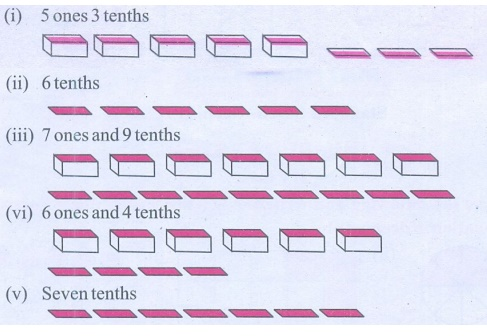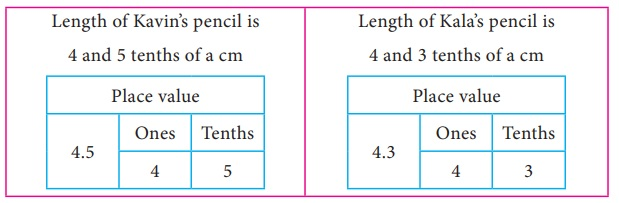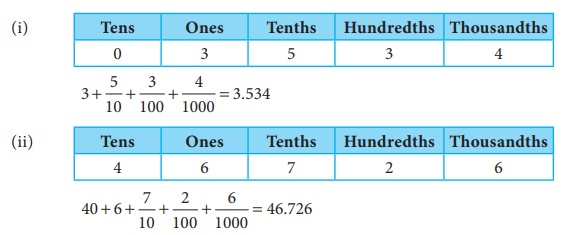Home | | Maths 7th Std | Representing a Decimal Number

# Representing a Decimal Number

Represent the following decimal numbers pictorially.

Representing a Decimal Number

(i) Observe the following pictures which represent tens, ones and tenthsFor example, the decimal number 3.2 is 3 ones and 2 tenths that can be represented using the pictorial representations as follows.Similarly any decimal number can be represented as above

Try this

Represent the following decimal numbers pictorially.

(i) 5 ones and 3 tenths

(ii) 6 tenths

(iii) 7 ones and 9 tenths

(iv) 6 ones and 4 tenths

(v) Seven tenthsWe have studied about place value of digits of a number in primary classes. Now let us extend our learning of place values to decimal digits of a number. Let us recall the expanded form of a number. Consider the number 3768.

The expanded form of 3768 is given by 3 × 1000 + 7 × 100 + 6 × 10 + 8 .

Now, let us consider a decimal number 235.68

Expressing the decimal number in the expanded form, we get

235.68 = 200 + 30 + 5 + 6/10 + 8/100

=2×100 + 3×10 + 5×1 + 6×1/10 + 8 × 1/100

Think

The above expression can also be expressed in terms of powers of 10 as follows 235.68 = 2 × 102 + 3 × 101 + 5 × 100 + 6 × 101 + 8 × 102 . Hence, in any number, when we move towards right from one digit to the next, the place value of a digit is divided by 101.

Representing the two numbers 3768 and 25.6 in the place value grid, we getIn the beginning of this chapter, we came across the discussion about the length of the pencils of Kavin and Kala. Those lengths can also be represented in the place value grid as follows.We know that the digit to the right of ones place is known as tenths and the point is called decimal point which separates the integral part and decimal part of a number.

In the above situation, we have expressed numbers with one decimal digit in place value grid. Now to express numbers with two decimal digits in place value grid, let us consider the example already discussed in the introduction part.

Ravi has purchased dress materials for pongal festival. The length of the pant material is 4 m 75 cm. To express the centimetre in terms of metres, we proceed as follows.

We know that

100 cm = 1 m

1 cm = 1/100 m

Therefore one centimetre can be represented as one hundredth of a metre.

Similarly, 75 cm = 75/100 = 0.75 m

So the length of the pant material is 4 + 0.75 m

That is 4.75 m. It can be read as four and seventy five hundredth of a metre or four point seven five metres.

Note

The decimal digits of a number have to be read as separate digits.

Try these

1. Express the following decimal numbers in an expanded form and place value grid form.

(i) 56.78

The expanded form of 56.78 = 50+6 + 7/10 + 8/100

= 5 × 10 + 6 × 1 + 7 × 1/10 + 8 1/100

Place value grid form(ii) 123.32

Expanded form of 123.32 = 100 + 20 + 3 + 3/10 + 2/100

= 1 × 100 + 2 × 10 + 3 × 1 + 3 × 1/10 + 2 1/100

Place value grid(iii) 354.56

Expanded form of 354.56 = 300 + 50 + 4 + 5/10 + 6/100

= 3 × 100 + 5 × 10 + 4 × 1 + 5 × 1/10 + 6 1/100

Place value grid2. Express the following measurements in terms of metre and in decimal form. One is done for you.3. Write the following numbers in the place value grid and find the place value of the underlined digits.

(i) 36.37

Place value grid36.37

The place value of 3 in 36.37 is Tenth.

(ii) 267.06

Place value grid267.0 6

The place value of 6 in 267.06 is Hundredth.

(iii) 0.23

Place value grid0.2 3

The place value of 2 in 0.23 is Tenth.

(iv) 27.69

Place value grid27.69

The place value of 9 in 27.69 is Hundredth.

(v) 53.27

Place value grid53.27

The place value of 2 in 53.27 is Tenth.

Note that the tenth and hundredth place values of a number can be written as 1/ 10 and 1/100 respectively.

Example 1.1 Represent the following decimal numbers pictorially.

(i) 0.3

(ii) 3.6

(iii) 2.7

(iv) 11.4

SolutionExample 1.2 Write the following in the place value grid and find the place value of the underlined digits.

(i) 0.37

(ii) 2.73

(iii) 28.271

Solution(i) The place value of 7 in 0.37 is Hundredth.

(ii) The place value of 7 in 2.73 is Tenth.

(iii) The place value of 7 in 28.271 is Hundredth.

Example 1.3 The height of a person is 165 cm. Express this height in metre.

Solution

Since, 1 cm= 1/100 m = 0.01 m

Given, height of the person = 165 cm.

Hence, the height of the person = 165 /100 = 1.65 m.

Example 1.4

Praveen goes trekking with his friends. He has to record the distance in kilometres in his sports book. Can you help him?

The trekking record for four days are given below

(i) 4 m

(ii) 28 m

(iii) 537 m

(iv) 3983 m

Solution

Since, 1 m = 1/1000 km = 0.001 kmSo, Praveen's trekking record is 0.004 km, 0.028 km, 0.537 km, 3.983 km.

Example 1.5

Express the numbers given in expanded form in the place value grid. Also write its decimal representation.SolutionTags : Number System | Term 2 Chapter 1 | 7th Maths , 7th Maths : Term 2 Unit 1 : Number System
Study Material, Lecturing Notes, Assignment, Reference, Wiki description explanation, brief detail
7th Maths : Term 2 Unit 1 : Number System : Representing a Decimal Number | Number System | Term 2 Chapter 1 | 7th Maths